# Power-Reducing Formulas and How to Use Them (With Examples)

• Author:
• Updated date:

Ray is a licensed engineer in the Philippines. He loves to write about mathematics and civil engineering.Learn about power-reducing formulas. Photo by Antoine Dautry on Unsplash

## Power-Reducing Formula

The power-reducing formula is an identity useful in rewriting trigonometric functions raised to powers. These identities are rearranged double-angle identities that function much like the double-angle and half-angle formulas.

Power-reducing identities in calculus are useful in simplifying equations that contain trigonometric powers resulting in reduced expressions without the exponent. Reducing the power of the trigonometric equations gives more space to understand the relationship between the function and its rate of change every single time. Any trig function such as sine, cosine, tangent, or their inverses may be raised to any power.

For instance, the given problem is a trigonometric function raised to the fourth power or higher; it can apply the power-reducing formula more than once to eliminate all the exponents until fully reduced.

### Power-Reducing Formulas for Squares

sin2 (u) = (1 – cos (2u)) / 2

cos2 (u) = (1 + cos (2u)) / 2

tan2 (u) = (1 – cos (2u)) / (1 + cos (2u))

### Power-Reducing Formulas for Cubes

sin3 (u) = (3sin (u) – sin (3u)) / 4

cos3 (u) = (3cos (u) – cos (3u)) / 4

tan3 (u) = (3sin (u) – sin (3u)) / (3cos (u) – cos (3u))

### Power-Reducing Formulas for Fourths

sin4 (u) = [3 – 4 cos (2u) + cos (4u)] / 8

cos4 (u) = [3 + 4 cos (2u) + cos (4u)] / 8

tan4 (u) = [3 – 4 cos (2u) + cos (4u)] / [3 + 4 cos (2u) + cos (4u)]

### Power-Reducing Formulas for Fifths

sin5 (u) = [10 sin (u) – 5 sin (3u) + sin (5u)] / 16

cos5 (u) = [10 cos (u) + 5 cos (3u) + cos (5u)] / 16

Scroll to Continue

## Read More From Owlcation

tan5 (u) = [10 sin (u) – 5 sin (3u) + sin (5u)] / [10 cos (u) + 5 cos (3u) + cos (5u)]

### Special Power-Reducing Formulas

sin2 (u) cos2 (u) = (1 – cos (4u)) / 8

sin3 (u) cos3 (u) = (3 sin (2u) – sin (6u)) / 32

sin4 (u) cos4 (u) = (3 - 4 cos (4u) + cos (8u)) / 128

sin5 (u) cos5 (u) = (10 sin (2u) - 5 sin (6u) + sin (10u)) / 512

## Power-Reducing Formula Proof

The power reduction formulas are further derivations of the double angle, half-angle, and the Pythagorean Identify. Recall the Pythagorean equation shown below.

sin2 (u) + cos2 (u) = 1

Let us first prove the power reducing formula for sine. Recall that the double angle formula cos (2u) is equal to 2 cos2 (u) – 1.

(1 – cos 2u) / 2 = [1 – (2 cos2 (u) – 1)] / 2

(1 – cos 2u) / 2 = [2(1 - cos2 (u)] / 2

(1 – cos 2u) / 2 = 1 – cos2 (u)

1 – cos2 (u) = sin2 (u)

Next, let us prove the power-reducing formula for cosine, still considering that the double angle formula cos (2u) is equal to 2 cos2 (u) – 1.

(1 + cos 2u) / 2 = [1 + (2 cos2 (u) – 1)] / 2

(1 + cos 2u) / 2 = [2(cos2 (u))] / 2

(1 + cos 2u) / 2 = cos2 (u)

## Example 1

### Using Power-Reducing Formulas for Sine Functions

Find the value of sin4x given that cos(2x) = 1/5.

### Solution

Since the given sine function has an exponent to the fourth power, express the equation sin4x as a squared term. It will be much easier to write the fourth power of the sine function in terms of squared power to avoid the use of the half-angle identities and double-angle identities.

sin4 (x) = (sin2x)2

sin4 (x) = ((1 – cos (2x))/2)2

Substitute the value of cos (2x) = 1/5 to the squared power reduction rule for the sine function. Then, simplify the equation to get the result.

sin4 (x) = ((1 – 1/5)/2)2

sin4 (x) = 4/25

The value of sin4x given that cos (2x) = 1/5 is 4/25.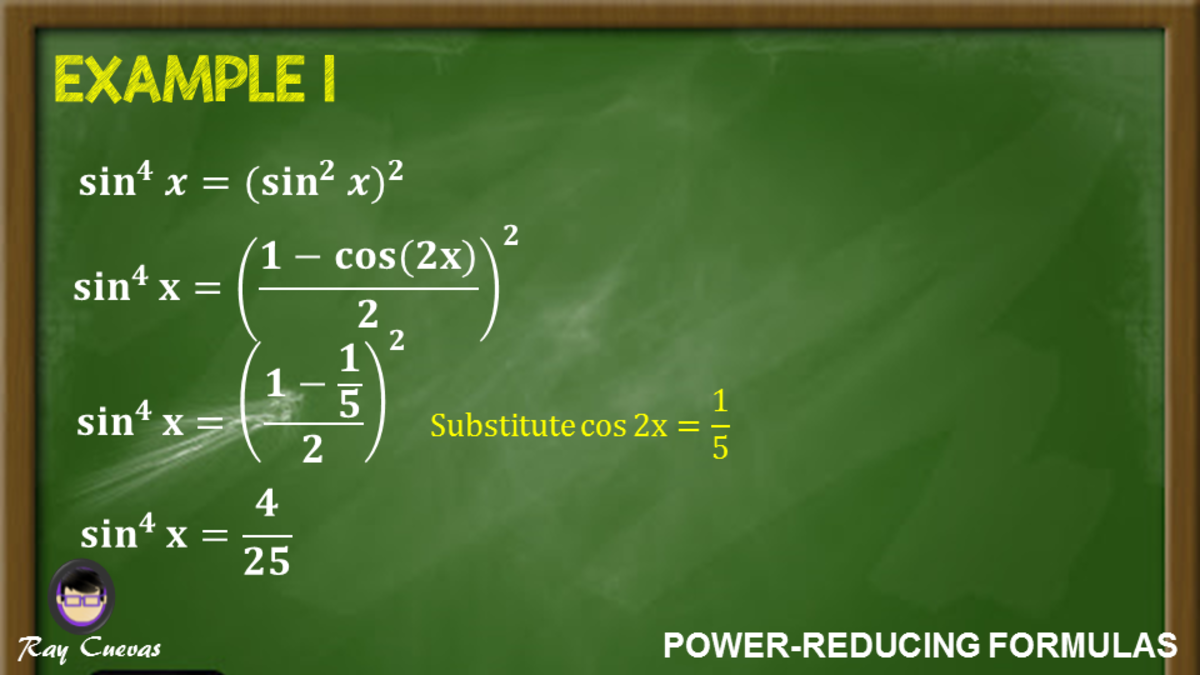Example 1: Using Power-Reducing Formulas for Sine FunctionsJohn Ray Cuevas

## Example 2

### Rewriting a Sine Equation to the Fourth Power Using the Power-Reducing Identities

Rewrite the sine function sin4x as an expression without powers larger than one. Express it in terms of the first power of the cosine.

Solution

Simplify the solution by writing the fourth power in terms of squared power. Although it can be expressed as (sin x)(sin x)(sin x)(sin x), but remember to retain at least a squared power in order to apply the identity.

sin4x = (sin2x)2

Use the power-reducing formula for cosine.

sin4x = ((1 – cos (2x))/2)2

sin4x = (1 – 2 cos (2x) + cos2 (2x)) / 4

Simplify the equation to its reduced form.

sin4x = (1/4) [1 – 2cos (2x) + (1 + cos (4x)/2)]

sin4x = (1/4) – (1/2) cos 2x + 1/8 + (1/8) cos 4x

sin4x = (3/8) – (1/2) cos 2x + (1/8) cos 4x

The reduced form of the equation sin4x is (3/8) – (1/2) cos 2x + (1/8) cos 4x.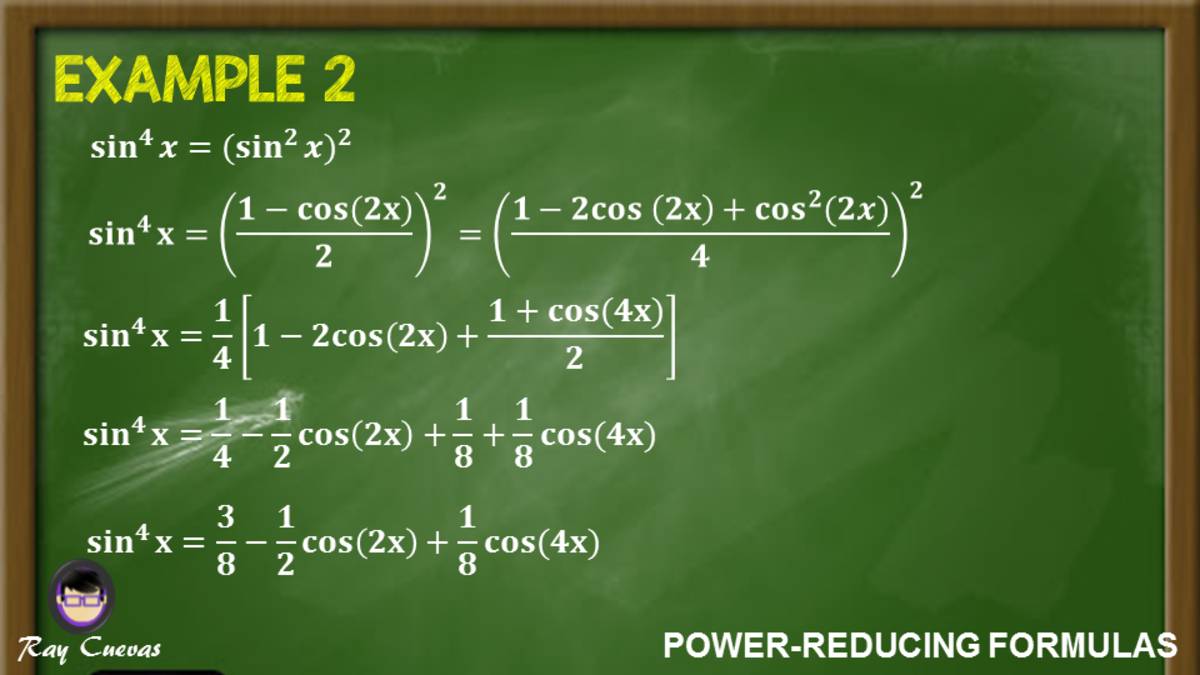Example 2: Rewriting a Sine Equation to the Fourth Power Using the Power-Reducing IdentitiesJohn Ray Cuevas

## Example 3: Simplifying Trigonometric Functions to the Fourth Power

Simplify the expression sin4 (x) – cos4 (x) using the power-reducing identities.

Solution

Simplify the expression by reducing the expression into square powers.

sin4 (x) – cos4 (x) = (sin2 (x) – cos2 (x)) (sin2 (x) + cos2 (x))

sin4 (x) – cos4 (x) = - (cos2 (x) – sin2 (x))

Apply the double angle identity for cosine.

sin4 (x) – cos4 (x) = - cos (2x)

The simplified expression of sin4 (x) – cos4 (x) is - cos (2x).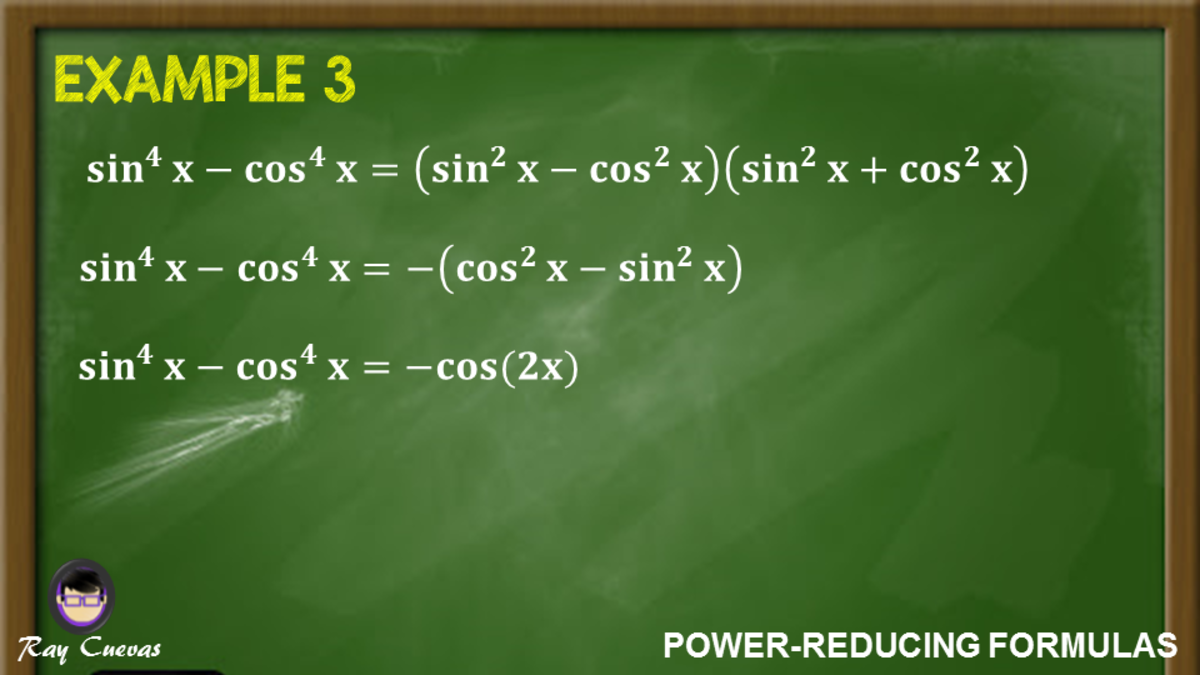Example 3: Simplifying Trigonometric Functions to the Fourth PowerJohn Ray Cuevas

## Example 4: Simplifying Equations to Sines and Cosines of First Power

Using the power-reduction identities, express the equation cos2 (θ) sin2 (θ) using only cosines and sines to the first power.

Solution

Apply the power-reducing formulas for cosine and sine, and multiply both of them. See the following solution below.

cos2θ sin2θ = cos2 (θ) sin2 (θ)

cos2θ sin2θ = (1/4) (2 cos θ sin θ)2

cos2θ sin2θ = (1/4) (sin2 (2θ))

cos2θ sin2θ = (1/4) [(1 – cos (4θ))/2]

cos2θ sin2θ = (1/8) [1 – cos (4θ)]

Therefore, cos2 (θ) sin2 (θ) = (1/8) [1 – cos (4θ)].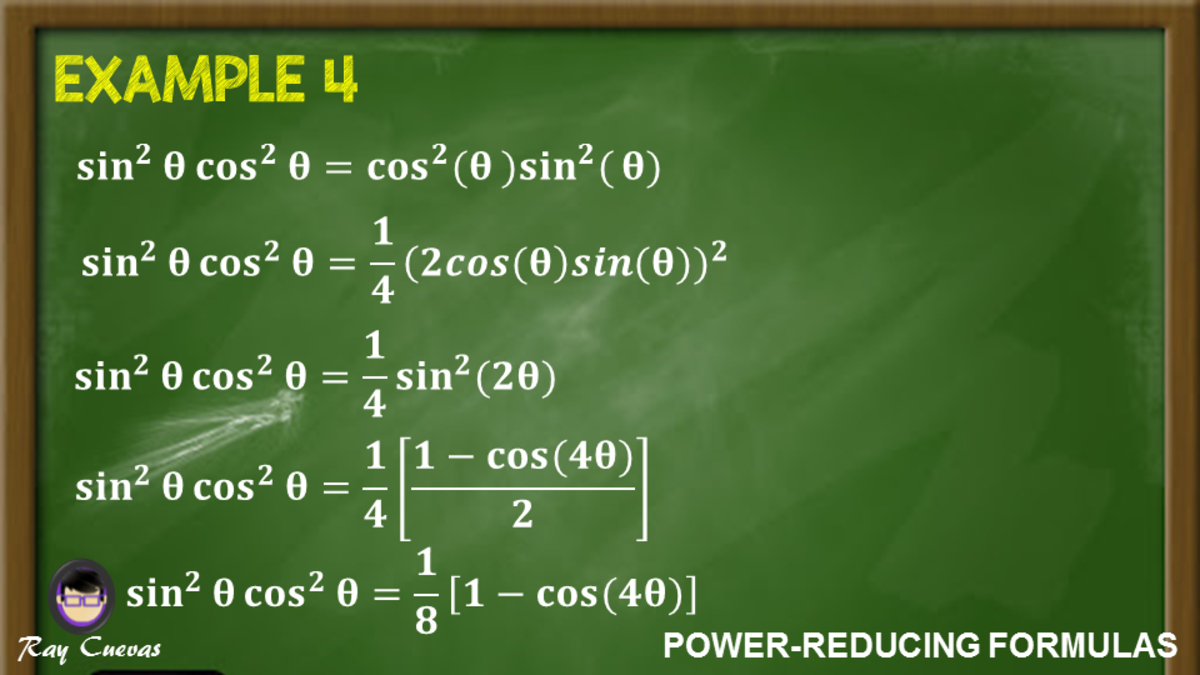Example 4: Simplifying Equations to Sines and Cosines of First PowerJohn Ray Cuevas

## Example 5: Proving the Power Reducing-Formula for Sine

Prove the power-reducing identity for sine.

sin2x = (1 – cos (2x)) / 2

Solution

Start simplifying the double-angle identity for cosine. Remember that cos (2x) = cos2 (x) – sin2 (x).

cos (2x) = cos2 (x) – sin2 (x)

cos (2x) = (1 – sin2 (x)) – sin2 (x)

cos (2x) = 1 – 2 sin2 (x)

Use the double-angle identity to simplify sin2 (2x). Transpose 2 sin2 (x) to the left equation.

2 sin2 (x) = 1 – cos (2x)

sin2 (x) = [(1 – cos (2x)) / 2]

Therefore, sin2 (x) = [(1 – cos (2x)) / 2].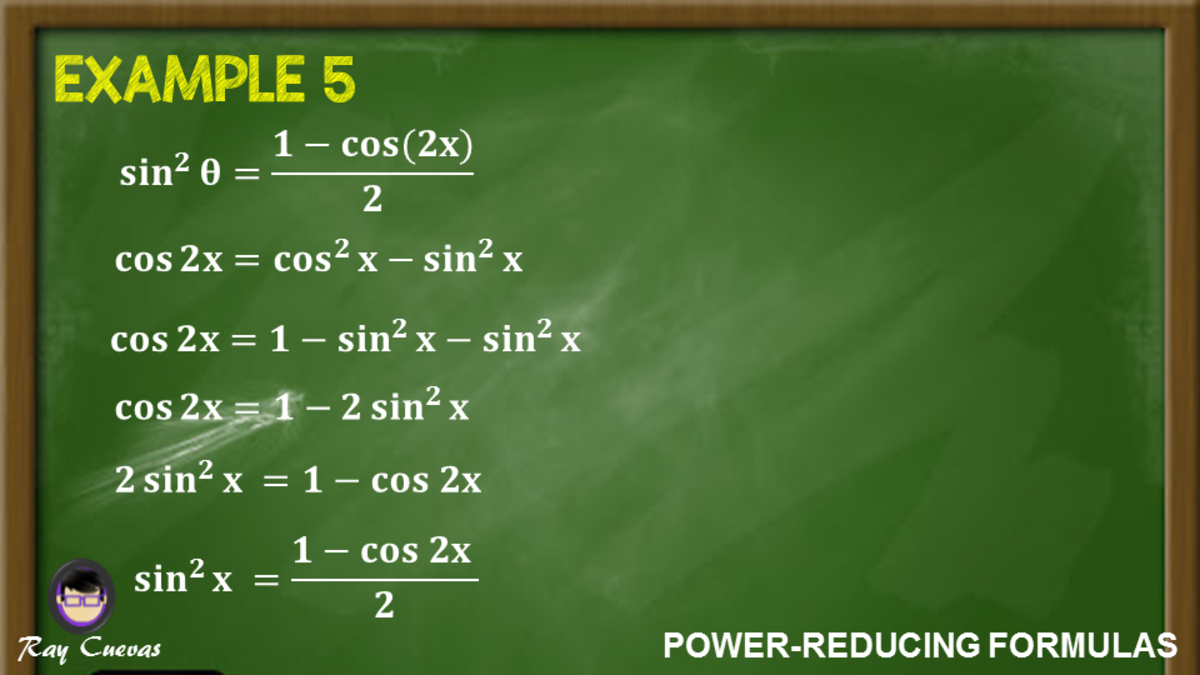Example 5: Proving the Power-Reducing Formula for SineJohn Ray Cuevas

## Example 6: Solving the Value of a Sine Function Using Power-Reducing Formula

Solve the sine function sin2 (25°) using the power-reducing identity for sine.

Solution

Recall the power-reducing formula for sine. Then, substitute the value of the angle measure u = 25° to the equation.

sin2 (x) = [(1 – cos (2x)) / 2]

sin2 (25°) = [(1 – cos (2(25°))) / 2]

Simplify the equation and solve for the resulting value.

sin2 (25°) = [(1 – cos (50°)) / 2]

sin2 (25°) = 0.1786

The value of sin2 (25°) is 0.1786.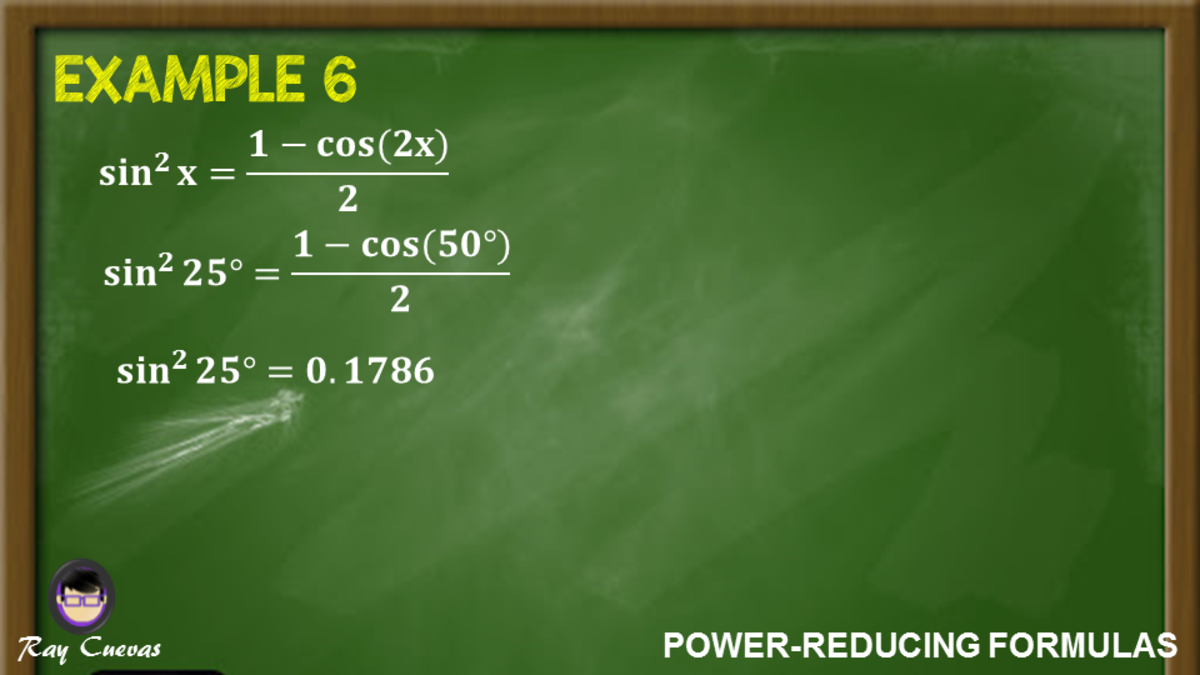Example 6: Solving the Value of a Sine Function Using Power-Reducing FormulaJohn Ray Cuevas

## Example 7: Expressing the Fourth Power of Cosine to the First Power

Express the power-reducing identity cos4 (θ) using only sines and cosines to the first power.

Solution

Apply the formula for cos2 (θ) two times. Consider θ as x.

cos4 (θ) = (cos2 (θ))2

cos4 (θ) = ([(1 + cos (2θ)]/2)2

Square both the numerator and the denominator. Use the power-reducing formula for cos2 (θ) with θ = 2x.

cos4 (θ) = [(1 + 2 cos (2θ) + cos2 (2θ)] / 4

cos4 (θ) = [(1 + 2 cos (2θ) + [1 + cos (4θ)/2]] / 4

cos4 (θ) = [(2 + 4 cos (2θ) + 1 + cos (4θ)] / 8

Simplify the equation and distribute 1/8 through the parentheses

cos4 (θ) = (1/8) [cos (4θ) + 4 cos (2θ) + 3)

cos4 (θ) = (1/8) cos (4θ) + (1/2) cos (2θ) + 3/8

The expression for cos4 (θ) with only sines and cosines to the first power is (1/8) cos (4θ) + (1/2) cos (2θ) + 3/8.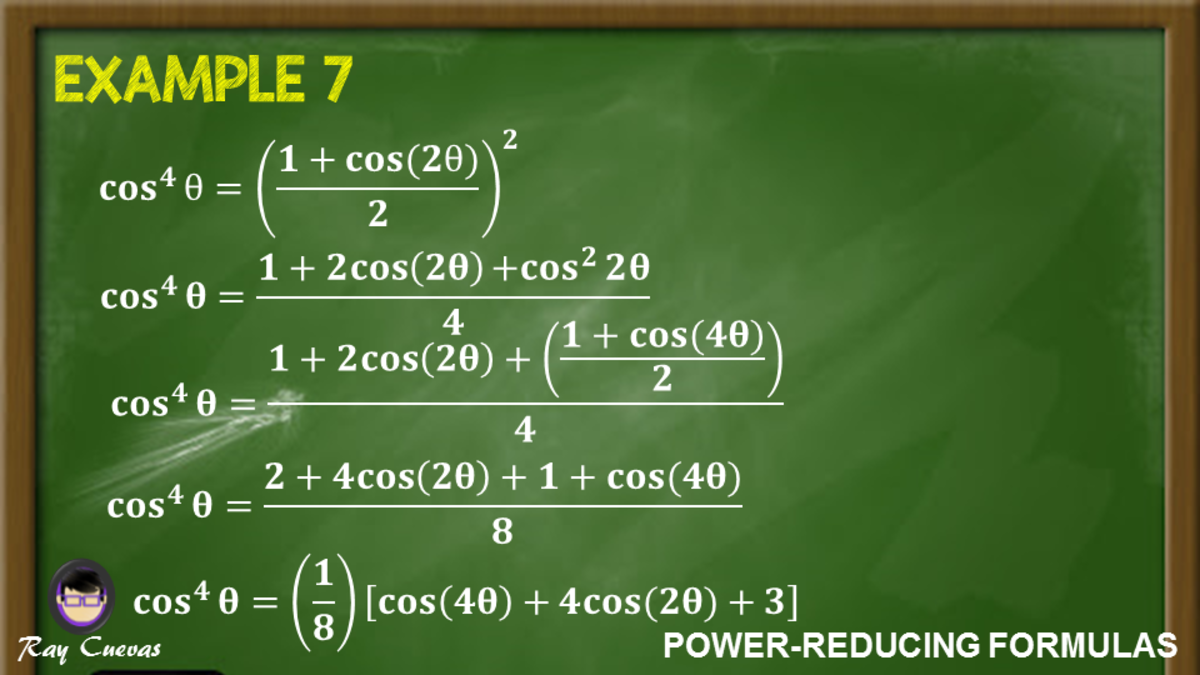Example 7: Expressing the Fourth Power of Cosine to the First PowerJohn Ray Cuevas

## Example 8: Proving Equations Using Power-Reducing Formula

Use the power-reducing formula to prove that 5 cos4 (x) = 15/8 + (5/2) cos (2x) + (5/8) cos (4x).

Solution

Rewrite the equation and apply the formula for cos2 (x) two times. Consider θ as x.

5 cos4 (x) = 5 (cos2 (x))2

Substitute the reduction formula for cos2 (x). Raise both the denominator and numerator the dual power.

5 cos4 (x) = 5 [(1 + cos (2x)) / 2]2

5 cos4 (x) = (5/4) [1 + 2 cos (2x) + cos2 (2x)]

Substitute the power-reducing formula of cosine to the last term of the resulting equation.

5 cos4 (x) = (5/4) + (5/2) cos (2x) + (5/4) [(1 + cos 2 (2x)) / 2]

5 cos4 (x) = (5/4) + (5/2) cos (2x) + (5/8) + (5/8) cos (4x)

5 cos4 (x) = 15/8 + (5/2) cos (2x) + (5/8) cos (4x)

Therefore, 5 cos4 (x) = 15/8 + (5/2) cos (2x) + (5/8) cos (4x).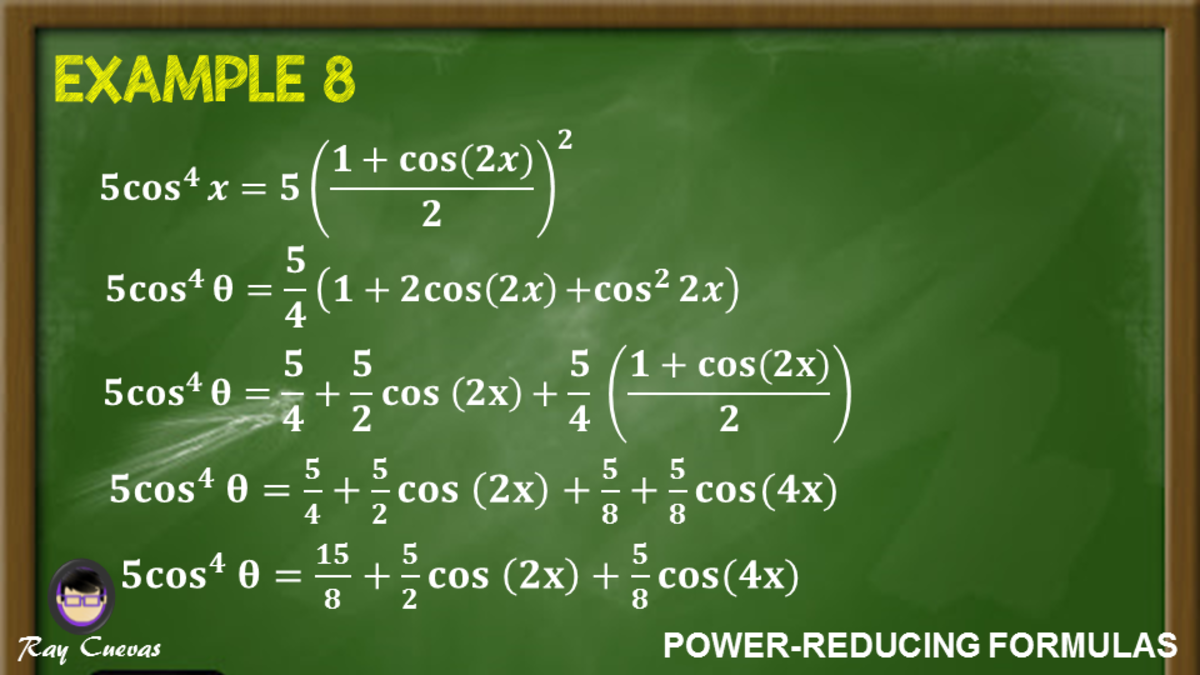Example 8: Proving Equations Using Power-Reducing FormulaJohn Ray Cuevas

## Example 9: Proving Identities Using the Power-Reducing Formula for Sine

Prove that sin3 (3x) = (1/2) [sin (3x)] [1 – cos (3x)].

Solution

Since the trigonometric function is raised to the third power, there will be one quantity of square power. Rearrange the expression and multiply one square power to a single power.

sin3 (3x) = [sin (3x)] [sin2 (3x)]

Substitute the power-reduction formula to the obtained equation.

sin3 (3x) = [sin (3x)] [(1 – cos (3x))/2]

Simplify to its reduced form.

sin3 (3x) = sin (3x) (1/2) (1 – cos (3x))

sin3 (3x) = (1/2) [sin (3x)] [1 – cos (3x)]

Therefore, sin3 (3x) = (1/2) [sin (3x)] [1 – cos (3x)].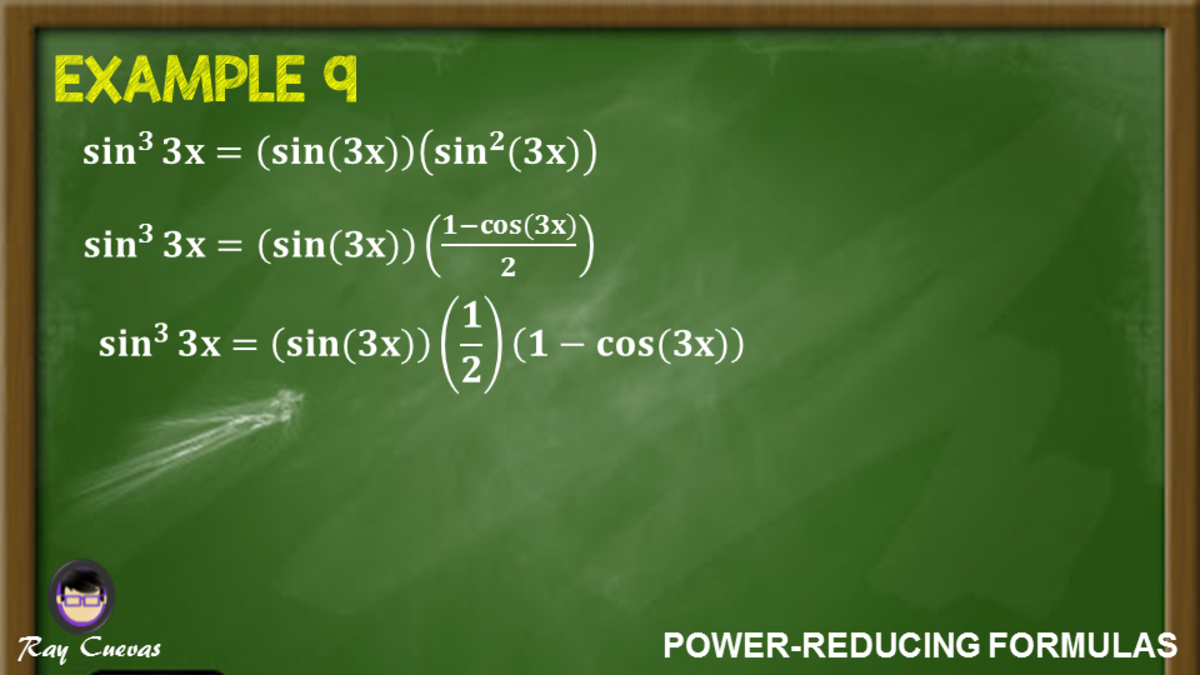Example 9: Proving Identities Using the Power-Reducing Formula for SineJohn Ray Cuevas

## Example 10: Rewriting a Trigonometric Expression Using the Power-Reducing Formula

Rewrite the trigonometric equation 6sin4 (x) as an equivalent equation having no powers of functions larger than 1.

Solution

Start rewriting sin2 (x) to another power. Apply the power-reduction formula two times.

6 sin4 (x) = 6 [sin2 (x)]2

Substitute the power-reducing formula for sin2 (x).

6 sin4 (x) = 6 [(1 – cos (2x)) / 2]2

Simplify the equation by multiplying and distributing constant 3/2.

6 sin4 (x) = 6 [(1 – 2 cos (2x) + cos2 (2x))] / 4

6 sin4 (x) = (3/2) [1 – 2 cos (2x) + cos2 (2x)]

6 sin4 (x) = (3/2) – 3 cos (2x) + (3/2) cos2 (2x)